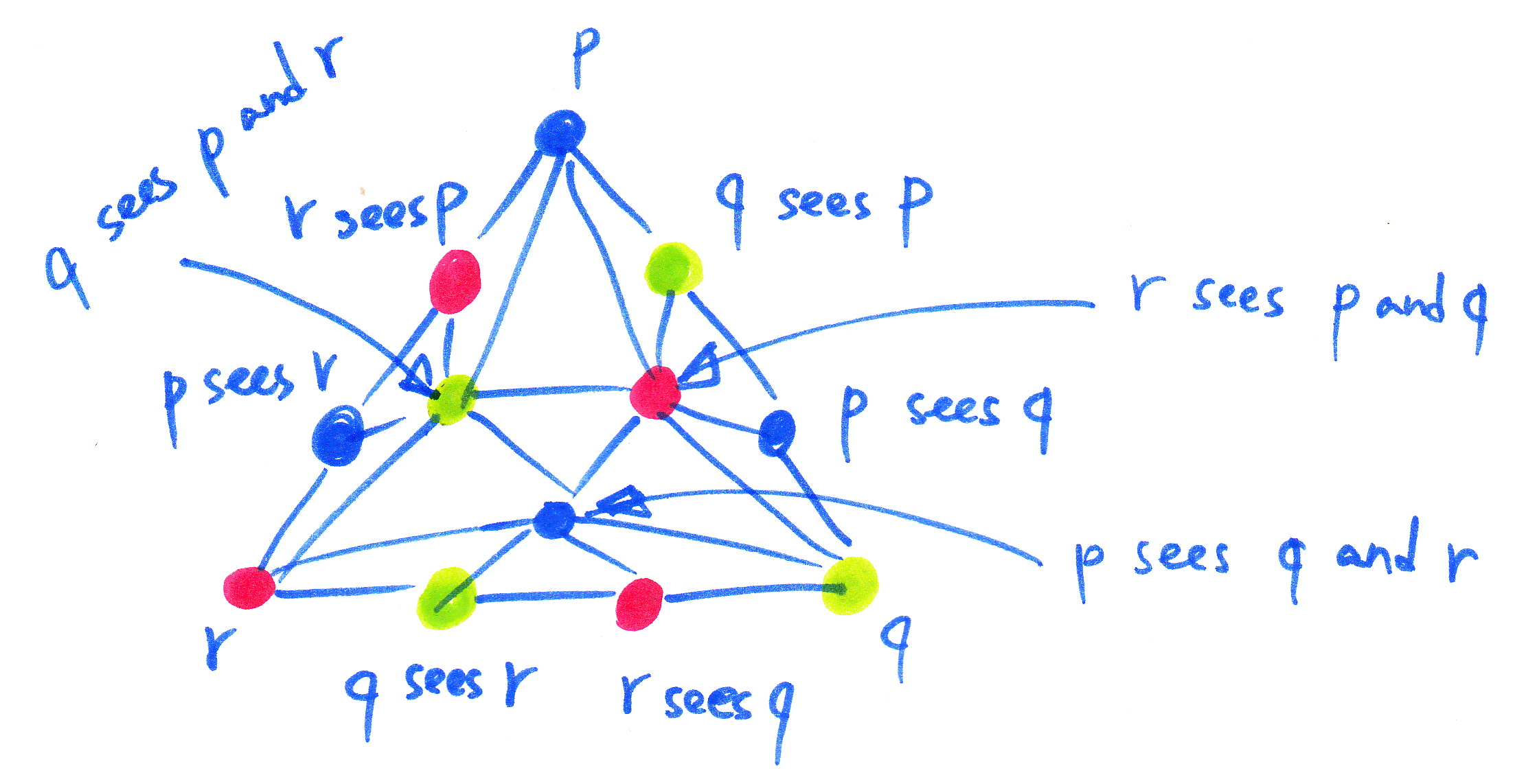# Mathematics for Efficient and Robust Distributed Computing

Goals:
Explore the potential of combinatorial topological in application to distributed computing.

Tools:
Logic, algorithmic reasoning, programming.

Prerequisites:
Maturity in math and algorithms, curiosity and adventurism, but some rigor too.

Practically all computing systems, from fire alarms to Internet-scale services, are nowadays distributed: they consist of a number of computing units performing independent computations and communicating with each other to synchronize their activities. Our dependence on performance and reliability of the distributed computing becomes more and more imminent. Therefore, understanding fundamentals of distributed computing is of crucial importance.

The main complication here is the existing immense diversity of distributed applications, models of distributed computations, and performance metrics, combined with the lack of mathematical tools to handle this complexity.

Recently, an impressive attempt to address this challenge was made: some long-standing open questions in distributed computability were resolved using advanced branches of modern mathematics, such as combinatorial and algebraic topology. More precisely, a set of possible concurrent executions can be represented as a geometrical structure, called simplicial complex, and all possible ways the concurrent system can evolve can be seen as a transformation of the simplicial complex in space.

For example, it turns out that the simplicial complex modelling the reachable states of a wait-free system (imposing neither synchrony assumptions nor bounds on the number of failures) is always contractible (connected in all dimensions) and, thus, there is no way to solve non-trivial set agreement (imposing an odd number of ``holes'') [2,4,1,3].However, most of the existing applications of topology in distributed computing concern theoretical positive or negative results, i.e., proving that no solution to a given problem in a given model exists or proving the existence fact in a non-constructive way. With a few exceptions, there are no convincing examples of using advanced mathematical tools to design new efficient algorithms.

At a higher level, this project aims at better understanding of what can and what cannot be implemented in specific distributed environments. In particular, we intend to apply the power of modern mathematics in deriving new algorithms and tight lower bounds for distributed computing problems.

The project (not exhaustively) consists of the following directions:

(1) Mathematical models for distributed computing.

We aim at developing a ``universal language'' to describe models of distributed computation. The language should be general enough to encompass realistic distributed systems and simple enough to make reasoning about these systems tractable.

(2) Distributed Computability

A crucial question in distributed computing is how to distinguish solvable from unsolvable, starting from one-shot problems, such as consensus or leader election, to long-lived abstractions, such as queues, dictionaries, or transactional memories.

(3) Protocol Design

Finally, once the line between solvable and unsolvable problems in a given models is demarcated, we should be able to infer concrete (and efficient!) distributed algorithms for solvable problems. The belief here is that formal and rigorous treatment of computing systems will help devising a methodology of building optimal distributed algorithms in a uniform way.

## Bibliography

 E. Borowsky and E. Gafni.
Generalized FLP impossibility result for -resilient asynchronous computations.
In STOC, pages 91-100. ACM Press, May 1993.

 M. Herlihy and N. Shavit.
The asynchronous computability theorem for -resilient tasks.
In STOC, pages 111-120, May 1993.

 M. Herlihy and N. Shavit.
The topological structure of asynchronous computability.
J. ACM, 46(2):858-923, 1999.

 M. Saks and F. Zaharoglou.
Wait-free -set agreement is impossible: The topology of public knowledge.
In STOC, pages 101-110. ACM Press, May 1993.